Select Page

# MCQ Answers for CBSE 12 Science Maths Linear Programming in English

MCQ Answers for CBSE 12 Science Maths Linear Programming in English to enable students to get Answers in a narrative video format for the specific question.

Expert Teacher provides MCQ Answers for CBSE 12 Science Maths Linear Programming through Video Answers in English language. This video solution will be useful for students to understand how to write an answer in exam in order to score more marks. This teacher uses a narrative style for a question from Linear Programming not only to explain the proper method of answering question, but deriving right answer too.

Please find the question below and view the Answer in a narrative video format.

Question:

## Similar Questions from CBSE, 12th Science, Maths, Linear Programming

Question 1 : The objective function is maximum or minimum, which lies on the boundary of the feasible region. (View Answer Video)

### Application of Integrals

Question 1 : Using integration, find the area of the region bounded by the curves: y = |x + 1| + 1, x = -3, x = 3, y = 0. (View Answer Video)

Question 2 : Find the area enclosed by the parabola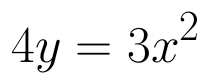and the line 2y = 3x + 12.   (View Answer Video)

Question 3 : Find the area bounded by the curve y = sin x between x = 0 and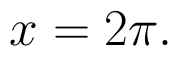(View Answer Video)

Question 4 : Using the method of integration, find the area of the region bounded by the lines 3x - 2y + 1 = 0, 2x + 3y -21 = 0 and x - 5y + 9 = 0. (View Answer Video)

Question 5 : Find the area between the curves y = x and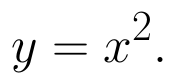(View Answer Video)

### Relations and Functions

Question 1 : Number of binary operations on the set {a, b} are : (View Answer Video)

Question 2 : Let R be the relation in the set N given by :
R = {(a, b) : a = b - 2, b > 6}.

Question 3 :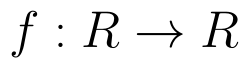be  defined as f(x) =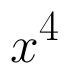(View Answer Video)

Question 4 :  If f is the greatest integer function and g is the modulus function . Write the value of g o f(-1/3) – f o g ( -1/3 ) . (View Answer Video)

Question 5 : Functions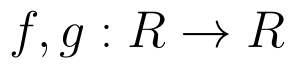are defined respectively, by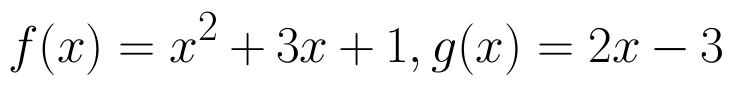, find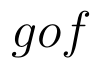(View Answer Video)

### Three Dimensional Geometry

Question 1 : Find the vector equation of the plane with intercepts 3, -4 and 2 on x, y and z-axis respectively. (View Answer Video)

Question 2 : Find the equation of plane passing through the line of intersection of the planes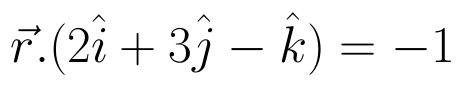and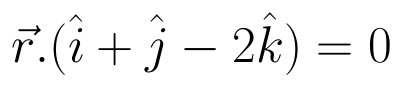and passing through the point (3, -2, -1). Also, find the angle between the two given planes. (View Answer Video)

Question 3 : Find the vector equation of a plane which is at a distance of 5 units from the origin and its normal vector is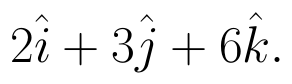(View Answer Video)

Question 4 : Find the distance of the point (1, -2, 3) from the plane x-y+z=5 measured parallel to the line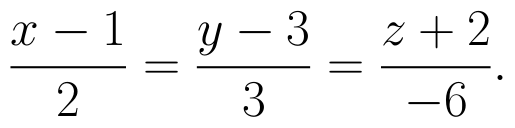(View Answer Video)

Question 5 : Find the cartesian equation of the line which passes through the point (-2, 4, -5) and is parallel to the line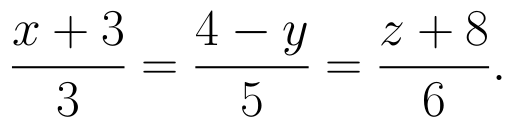(View Answer Video)